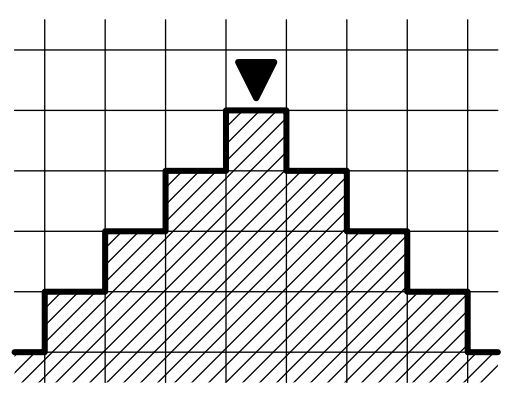시간 제한 메모리 제한 제출 정답 맞은 사람 정답 비율
1 초 512 MB 0 0 0 0.000%

## 문제

Mike is creating robots that would play hide-and-seek game. The game of hide-and-seek for robots will take place on a rectangular grid that has the size of $m \times n$, some of its cells are occupied with robots.

Each robot looks in one of the four directions: upwards, downwards, to the left, or to the right. Each robot has its view area. Consider a robot that looks downwards. His view area contains one cell in the following row, three cells in the row next to the following, five in the next row, etc. The $k$-th row has a segment of $2k - 1$ cells in the robot's view area, its center is in the same column as the robot.

The view areas of the robots looking to the right, upwards and to the left are defined analogously.The robot $A$ sees the robot $B$ if the cell where the robot $B$ is located at is in the view area of the robot $A$. In order to start the hide-and-seek game for robots there must be no pair of robots on the playfield that both see another one from the pair. That is, for each pair $A$, $B$ of robots at least one of two conditions must be satisfied: $A$ is not in the view area of the robot $B$, or $B$ is not in the view area of the robot $A$.

In one step Mike can turn any robot 90 degrees clockwise or counterclockwise. This operation changes the direction the robot is looking correspondingly. He would like to start the game as soon as possible, so he wants to make the minimum number of steps to get the configuration that allows to start the game.

Help him find such configuration. It can be proved that for any initial configuration of robots such configuration exists.

## 입력

The first line of input contains two integers $m$ and $n$, the number of rows and the number of columns of the grid ($1 \le n, m \le 2\,000$).

The following $m$ lines contain $n$ characters each, each character is one of 'U', 'D', 'L', 'R', or '.'. The character 'U' denotes the robot that looks upwards, the character 'D' --- the one that looks downwards, the character 'L' --- the one that looks to the left, the character 'R' --- the one that looks to the right, the character '.' means that this cell doesn't contain a robot.

## 출력

Output $m$ lines, each must contain $n$ characters --- the configuration that allows to start the game. The configuration must be obtained from the one given in input by minimum number of steps. If there are several possible answers, you can output any one.

Note that Mike can only turn robots, he cannot remove them nor add them to the play field. So the robots in the output must be exactly at the same positions as in the input.

## 예제 입력 1

2 3
RDL
.U.


## 예제 출력 1

UDL
.R.


## 예제 입력 2

2 2
..
..


## 예제 출력 2

..
..